# Pushing a box at an angle

chahud42

## Homework Statement

A force is applied to an initially stationary block of mass 2.65 kg that sits on a horizontal floor as shown. The 81.0 N force is applied at θ = 50° angle to the vertical. The coefficients of friction between the floor and the block are μs = 0.895 and μk = 0.665. What is the acceleration of the block?

## Homework Equations

Newton's second law and trig

## The Attempt at a Solution

First I broke the force into components, Fx=81sin50, Fy=81cos50. There are also the force of gravity, normal force, and friction acting on the block. So for sum of forces equations i have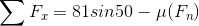, and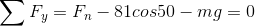. So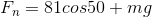. Knowing normal force, you can substitute that in the equation for x-axis.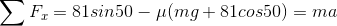. Next I wanted to figure out whether the acceleration is 0 because it doesn't overcome friction or not. So I compared the force of friction and applied force. Force of static friction I worked out to be 46.6N, where the applied force is 81sin50, which is 62N. So, we use kinetic friction....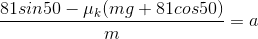Where did I go wrong????

scottdave
Homework Helper
How did you arrive at 46.6 Newtons for static force? What value is the normal force? Look back at your formula for Fn, did you forget something?

chahud42
How did you arrive at 46.6 Newtons for static force? What value is the normal force? Look back at your formula for Fn, did you forget something?
To find the static force I multiplied .895 by the expression for Fn. I took that as the force of friction to overcome, which the applied force of 62N overcomes, and accelerates the system so that kinetic friction must use. To find normal force, I applied newtons second law to the y-axis. ∑Fy=0 (acceleration is equal to 0). ∑Fy=Fg+Fappliedy-Fn=0
Sooooo Fn=Fg+Fapplied=mg+81cos50

Am I looking at something wrong?

haruspex
Homework Helper
Gold Member
2020 Award
To find the static force I multiplied .895 by the expression for Fn
Sure, but as scottdave hinted you forgot part of your equation when calculating the normal force.

chahud42
Sure, but as scottdave hinted you forgot part of your equation when calculating the normal force.
I genuinely have no clue. The only forces in the negative y direction are the force of gravity and the y component of the applied force. So the normal force should be equal to the sum of those.

ehild
Homework Helper
To find the static force I multiplied .895 by the expression for Fn. I took that as the force of friction to overcome, which the applied force of 62N overcomes, and accelerates the system so that kinetic friction must use. To find normal force, I applied newtons second law to the y-axis. ∑Fy=0 (acceleration is equal to 0). ∑Fy=Fg+Fappliedy-Fn=0
Sooooo Fn=Fg+Fapplied=mg+81cos50

Am I looking at something wrong?
What is the numerical value of the normal force?

haruspex
Homework Helper
Gold Member
2020 Award
I genuinely have no clue. The only forces in the negative y direction are the force of gravity and the y component of the applied force. So the normal force should be equal to the sum of those.
You initially wrote, correctly
And again post #3:
Sooooo Fn=Fg+Fapplied=mg+81cos50
But when you calculated ##\mu F_n## you appear to have forgotten the mg term. If you still cannot get the right answer, please post all working in complete detail.

chahud42
You initially wrote, correctly

And again post #3:

But when you calculated ##\mu F_n## you appear to have forgotten the mg term. If you still cannot get the right answer, please post all working in complete detail.
I re-crunched the numbers and got 69.84N for the force of static friction and 62.05 for the applied force, so it seems it doesn't overcome it at all, making the acceleration zero. I can't believe I had it right the whole time. I must have just had it written down without the mg term in my notebook. Thanks for the help.